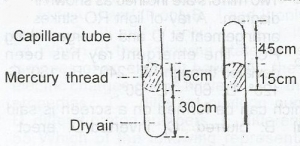# The set-up illustrated above shows a capillary tube of uniform cross-sectional area in two different arrangements. Using the data in the diagrams. Calculate...

Question 1The set-up illustrated above shows a capillary tube of uniform cross-sectional area in two different arrangements. Using the data in the diagrams. Calculate the pressure of the atmosphere